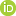This is a Preprint and has not been peer reviewed. The published version of this Preprint is available: https://doi.org/10.1093/gji/ggaa168. This is version 3 of this Preprint.

##### Downloads

Download Preprint

##### Authors

Frederik Tilmann, Hamzeh Sadeghisorkhani, Alexandra Mauerberger

##### Abstract

In probabilistic Bayesian inversions, data uncertainty is a crucial parameter for quantifying the uncertainties and correlations of the resulting model parameters or, in transdimensional approaches, even the complexity of the model. However, in many geophysical inference problems it is poorly known. Therefore, it is common practice to allow the data uncertainty itself to be a parameter to be determined. Although in principle any arbitrary uncertainty distribution can be assumed, Gaussian distributions whose standard deviation is then the unknown parameter to be estimated are the usual choice. In this special case, the paper demonstrates that a simple analytical integration is sufficient to marginalise out this uncertainty parameter, reducing the complexity of the model space without compromising the accuracy of the posterior model probability distribution. However, it is well known that the distribution of geophysical measurement errors, although superficially similar to a Gaussian distribution, typically contains more frequent samples along the tail of the distribution, so-called outliers. In linearised inversions these are often removed in subsequent iterations based on some threshold criterion, but in Markov chain Monte Carlo inversions this approach is not possible as they rely on the likelihood ratios, which cannot be formed if the number of data points varies between the steps of the Markov chain. The flexibility to define the data error probability distribution in Markov chain Monte Carlo can be exploited in order to account for this pattern of uncertainties in a natural way, without having to make arbitrary choices regarding residual thresholds. In particular, we can regard the data uncertainty distribution as a mixture between a Gaussian distribution, which represent valid measurements with some measurement error, and a uniform distribution, which represents invalid measurements. The relative balance between them is an unknown parameter to be estimated alongside the standard deviation of the Gauss distribution. For each data point, the algorithm can then assign a probability to be an outlier, and the influence of each data point will be effectively downgraded according to its probability to be an outlier. Furthermore, this assignment can change as the Markov chain Monte Carlo search is exploring different parts of the model space. The approach is demonstrated with both synthetic and real tomography examples. In a synthetic test, the proposed mixed measurement error distribution allows recovery of the underlying model even in the presence of 6% outliers, which completely destroy the ability of a regular Markov chain Monte Carlo or linear search to provide a meaningful image. Applied to an actual ambient noise tomography study based on automatically picked dispersion curves, the resulting model is shown to be much more consistent for different data sets, which differ in the applied quality criteria, while retaining the ability to recover strong anomalies in selected parts of the model.

##### DOI

https://doi.org/10.31223/osf.io/bcs4j

##### Subjects

Earth Sciences, Geophysics and Seismology, Physical Sciences and Mathematics

##### Keywords

Bayesian inversion, Data Uncertainty, Markov chain Monte-Carlo

##### Dates

Published: 2019-10-25 11:58

Last Updated: 2020-05-22 23:45

##### License

CC BY Attribution 4.0 International

Add a Comment

You must log in to post a comment.

Comments

There are no comments or no comments have been made public for this article.